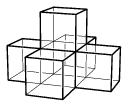Mathematical and Physical Journal
for High Schools
Issued by the MATFUND Foundation
 Already signed up? New to KöMaL?

# KöMaL Problems in Mathematics, May 2009

Show/hide problems of signs:## Problems with sign 'C'

Deadline expired on June 15, 2009.

C. 990. Find all integers for which the value of the expression 6x2-167x-4823 is

a) a prime number;

b) the smallest possible integer.

(5 pont)

solution (in Hungarian), statistics

C. 991. A right-angled triangle of sides 3, 4, 5 is cut into two parts with a line perpendicular to the hypotenuse. One part is a quadrilateral that has an inscribed circle, and the other part is a right-angled triangle. Find the lengths of the sides of the quadrilateral.

(5 pont)

solution (in Hungarian), statistics

C. 992. To boost the sales of a certain kind of sweets, the manufacturing company encloses gift vouchers in some of the boxes. The managers consider that the campaign will be efficient and costs will be bearable if the probability for a customer buying 10 boxes of sweets to find at least 1 voucher is about 50%. One in how many boxes should contain a voucher to achieve that?

(5 pont)

solution (in Hungarian), statistics

C. 993. The midpoint of the base AB of an isosceles triangle ABC is F. Let D denote the orthogonal projection of point F onto side BC. Let E be the midpoint of the line segment DF. Prove that CE is perpendicular to AD.

(5 pont)

solution (in Hungarian), statistics

C. 994. Let x<y<z. Solve the following equation on the set of natural numbers:.

(5 pont)

solution (in Hungarian), statistics## Problems with sign 'B'

Deadline expired on June 15, 2009.

B. 4182. The endpoints of the hypotenuse of a right-angled triangle of area t are the foci of an ellipse, and the third vertex lies on the ellipse. Prove that the area of the ellipse is at least.

(3 pont)

solution (in Hungarian), statistics

B. 4183. Find those arithmetic progressions in which the sum of the first n elements is a perfect square for every n.

(4 pont)

solution (in Hungarian), statistics

B. 4184. The vertices of a cyclic quadrilateral divide the circumscribed circle into four arcs. The midpoints of the arcs are, in this order, F1, F2, F3 and F4. Show that the line segment F1F3 is perpendicular to F2F4.

(3 pont)

solution (in Hungarian), statistics

B. 4185. Show that every non-zero polynomial can be multiplied by an appropriate non-zero polynomial, such that the exponent in each term of the product polynomial is divisible by 3.

(5 pont)

solution (in Hungarian), statistics

B. 4186. In an acute-angled triangle ABC, circles are drawn around the altitudes as diameters. In each circle, a chord is drawn that is perpendicular to the appropriate altitude and passes through the orthocentre of the triangle. Prove that the three chords obtained in this way are equal in length.

(3 pont)

solution (in Hungarian), statistics

B. 4187. Let a, k and n be positive integers. Find the greatest common divisor of the numbers an+1 and ak+1.

(5 pont)

solution (in Hungarian), statistics

B. 4188. Sides AB and CD of a quadrilateral ABCD are not parallel. Let E and F, respectively, be interior points of these sides. Prove that the midpoints of the line segments AF, CE, BF and DE form a convex quadrilateral whose area is independent of the choice of points E and F.

Kvant

(4 pont)

solution (in Hungarian), statistics

B. 4189. Determine all monotonic functions f defined on the set of real numbers, such that f(x)+2x=f(f(x)) for all real x.

Kvant

(5 pont)

solution (in Hungarian), statistics

B. 4190. The solid shown in the Figure is made up of six congruent cubes glued together. Is it possible to fill the space without gaps and overlaps with congruent copies of this solid?(5 pont)

solution (in Hungarian), statistics

B. 4191. Find all positive integers a, b, c for which 2a-1 is divisible by b, 2b-1 is divisible by c and 2c-1 is divisible by a.

(5 pont)

solution (in Hungarian), statistics## Problems with sign 'A'

Deadline expired on June 15, 2009.

A. 482. Let n be a positive integer. Prove that.

(5 pont)

solution, statistics

A. 483. For abritrary integers 0<kn and a>1, define.

(a) Show thatis an integer.

(b) Do there exist integers 0<k<n<m and a>1, for whichdivides?

(5 pont)

statistics

A. 484. In the Cartesian plane, a convex polygonal disk K is given. Apply the perpendicular affinity (x,y)(x,py) to the points of K, where 0<p<1. Denote the resulting polygonal disk by K'. Prove that K' can be covered by a polygonal disk which is congruent to K.

(5 pont)

solution, statistics